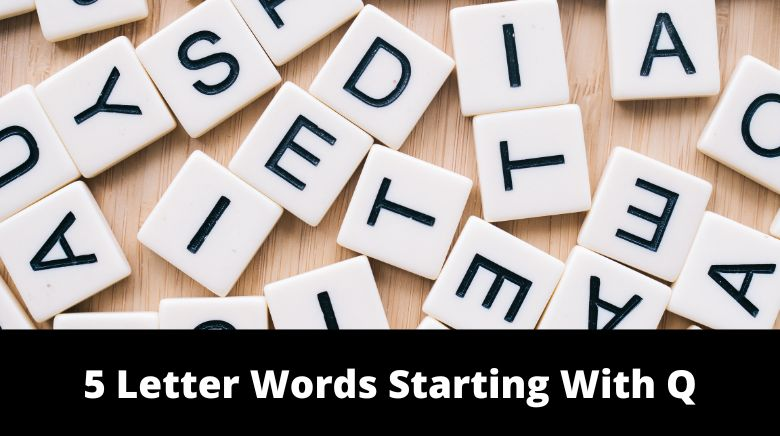# 5 Letter Words Starting With Q

“5 Letter Words Starting With Q”. A list of all five letter words that start with the letter Q.“5 Letter Words Starting With Q”

Below we have listed all the “five-letter words” that meet the criteria of your query;

• Word Limit: Five Letters
• Must Have This Letter(First Position): “Q”

Five letter words that start with the letter “Q.” The list we have shared below should feature all the words in the English dictionary that meet the criteria mentioned above. But if you know more, please do us a favor by sharing it in the comment box below.

Contents

## “5 Letter Words Starting With Q”

These are the five letter words that start with the letter Q.

• qaids
• qajaq
• qanat
• qapik
• qibla
• qilas
• qipao
• qophs
• qorma
• quabs
• quack
• quaff
• quags
• quail
• quair
• quais
• quake
• quaky
• quale
• qualm
• qualy
• quank
• quant
• quare
• quark
• quarl
• quart
• quash
• quasi
• quass
• quate
• quats
• quawk
• quaws
• quayd
• quays
• qubit
• quean
• queck
• queek
• queem
• queen
• queer
• quell
• queme
• quena
• quern
• query
• queso
• quest
• quete
• queue
• queyn
• queys
• queyu
• quibs
• quich
• quick
• quids
• quies
• quiet
• quiff
• quila
• quill
• quilt
• quims
• quina
• quine
• quink
• quino
• quins
• quint
• quipo
• quips
• quipu
• quire
• quirk
• quirl
• quirt
• quist
• quite
• quits
• quods
• quoif
• quoin
• quois
• quoit
• quoll
• quonk
• quops
• quork
• quorl
• quota
• quote
• quoth
• quouk
• quoys
• quran
• qursh
• quyte

“5 Letter Words Starting With Q”